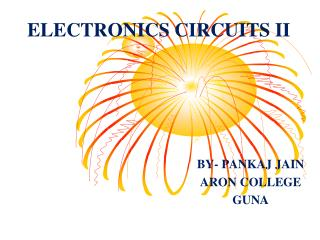DownloadDownload PresentationELECTRONICS CIRCUITS II

# ELECTRONICS CIRCUITS II

Download Presentation## ELECTRONICS CIRCUITS II

- - - - - - - - - - - - - - - - - - - - - - - - - - - E N D - - - - - - - - - - - - - - - - - - - - - - - - - - -
##### Presentation Transcript

1. ELECTRONICS CIRCUITS II BY- PANKAJ JAIN ARON COLLEGE GUNA

2. RC COUPLED AMPLIFIER

3. RC COUPLED AMPLIFIER • RC coupled amplifier is a common emitter transistor amplifier configuration. • RC coupled amplifier can be designed by using potential divider biasing circuit. • RC coupled amplifier can be used as voltage amplifier. • The o/p of RC coupled amplifier is simply taken from collector. • The coupling used is RC coupling.

4. RC COUPLED AMPLIFIER (Cntd..) • RC coupled amplifier is a medium frequency amplifier. • R1,R2 combination provides the base current. • RE is used for bias stabilization. • CE is used to by pass the ac components developed across RE. • RC is the collector resistor and it act as a load.

5. RC COUPLED AMPLIFIER(Cntd..) VCC • Circuit Diagram RC O/P R1 CC CC I/P CE R2 RE

6. RC COUPLED AMPLIFIER(Cntd..) • The voltage gain of RC coupled amplifier is grater than unity. • The Q point of the transistor must fix in the centre of the active region/Load line. • For that ,fix VCEQ=50% VCC and • IERE= 10% VCC ICRC= 40% VCC

7. RC COUPLED AMPLIFIER(Cntd..) • LOAD LINE VCC/(RC+RE) Q POINT ICQ VCEQ VCC

8. RC COUPLED AMPLIFIER(Cntd..) • h parameter equivalentcircuit • To draw the h parameter equivalent circuit, • Draw the AC behavior of the circuit • AC behavior of a circuit • Open circuit all capacitors. • Short circuit all DC power supply.

9. RC COUPLED AMPLIFIER(Cntd..) VCC I/P O/P

10. RC COUPLED AMPLIFIER(Cntd..) • AC behavior of a circuit R1 R2 RC

11. RC COUPLED AMPLIFIER(Cntd..) • h parameter equivalentcircuit hie hfeib + 1/hoe R1 hrevce RC R2 -

12. RC COUPLED AMPLIFIER(Cntd..) Simplified h parameter equivalentcircuit R1,R2>>hie so R1//R2//hie=hie hre Vce is a very small voltage , so it is neglected. 1/hoe//RC=RC Now ckt becomes

13. RC COUPLED AMPLIFIER(Cntd..) Simplified h parameter equivalentcircuit hie hfeib I/P RC

14. RC COUPLED AMPLIFIER(Cntd..) Frequency Response Gain BW f1 f1 Frequency

15. RC COUPLED AMPLIFIER(Cntd..) • If we express gain in db, frequency response will be Max Gain in db 3db Gain BW f1 Frequency f1

16. RC COUPLED AMPLIFIER(Cntd..) Design Assume VCC,IC,β O/P Loop equation VCC=ICRC +VCE+IERE VCE=50% VCC, ICRC=40% VCC Assume that Ic=IE IERE=10% VCC I/P Loop equation IC= β IB VCC=10 IB R1+VBE+IERE ; 9IB R2=VBE+IERE R1=VCC –VBE - IERE/ 10 IB ;R2=VBE+IERE/ 9IB

17. THANK YOU